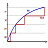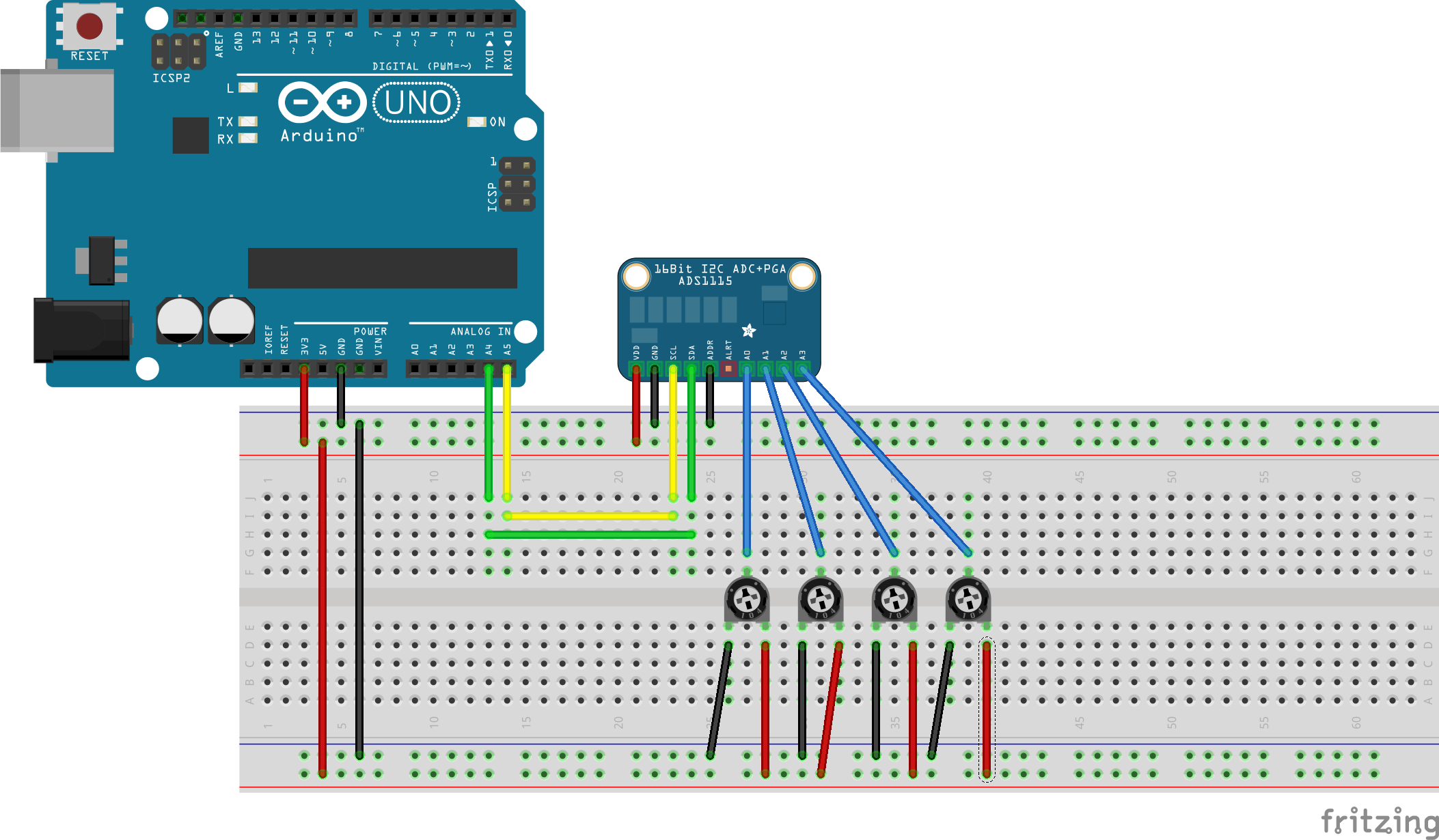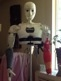javadoc

Ads1115 is a 4-channel A/D converter that you can communicate with using i2c.

It is supported in MRL using the Ads1115 service.

Go to Adafruit product page for more descriptions on details of board

Steps to control Servo Driver using the Arduino.

1. Connect the Arduino to the computer using a USB cable.

2. Connect the Ads1115 board to GND, VCC, DSA and SCL to the corresponding pins on the Arduino.

3. Connect the analog input to any of the 4 inputs A0-A3. In the example each input is connected to a trimpot so that the input can vary between 0 and 3.3 V.

4. Connect the Addr pin on the Ads1115 to GND for the default address of 0x48. It can also be connected to VCC (0x49), SDA (0x4A) or SCL ( 0x4B ) for different i2c addresses. That way up to four Ads115 can be connected to the same bus. If you want to connect more, you can use a i2c multiplexer.Use the example script below to measure all the four inputs.

You can use either the Arduino i2c pins or the Rasberry PI GPOI i2c pins. The example script has code for both alternatives.

```#
# Example code for Ads1115 4-channel AD converter on the i2c bus.
#
port = "COM3"
#
WebGui = Runtime.start("WebGui","WebGui")

if ('virtual' in globals() and virtual):
virtualArduino = Runtime.start("virtualArduino", "VirtualArduino")
virtualArduino.connect(port)

# This section is to be used if you use the i2c pins of the Arduino
arduino = Runtime.start("Arduino","Arduino")
arduino.connect(port)
# Sleep so that the Arduino can be initialized
sleep(4)

# This section is to be used if you use the i2c pins of the Raspberry PI
# raspi = Runtime.start("Raspi","RasPi")

# This section is common and shows how you can get the raw adc values and the voltages

```

## Examples:

```#
# Example code for Ads1115 4-channel AD converter on the i2c bus.
#
port = "COM3"
#
WebGui = Runtime.start("WebGui","WebGui")

if ('virtual' in globals() and virtual):
virtualArduino = Runtime.start("virtualArduino", "VirtualArduino")
virtualArduino.connect(port)

# This section is to be used if you use the i2c pins of the Arduino
arduino = Runtime.start("Arduino","Arduino")
arduino.connect(port)
# Sleep so that the Arduino can be initialized
sleep(4)

# This section is to be used if you use the i2c pins of the Raspberry PI
# raspi = Runtime.start("Raspi","RasPi")

# This section is common and shows how you can get the raw adc values and the voltages

```### Seem worky. PERFECT !

Seem worky. PERFECT !### Yes did not know it was in

Yes did not know it was in already! This is what Greg and myself were talking about in Eindhoven. To read the pot from the servo, and thus see where the servo is in real time. Now I can play with this sooner. Thought we didn't have the service in.

When I'm a bit further I'll make a blog post with data. Thanks